# Abstract

In this vignette we show how to detect quadratic phase coupling (QPC) of one-dimensional or multi-dimensional real-valued time series by bicoherence or cross_bicoherence of rhosa, respectively. The first section gives an example applying bicoherence to the data from a simple model exhibiting QPC at each frequency pair. In the second section we describe a generative model of three channels with another kind of QPC revealed by cross_bicoherence. The third section summarizes when and why bicoherence or cross_bicoherence fails to recognize a certain type of QPC.

# A toy model of QPC

We begin with importing rhosa:

library(rhosa)

Our first mathematical model, adapted from , is a superposition of six cosine curves of unit amplitude with different frequencies, named $$x_1$$:

$x_1(t) = \sum_{i=1}^{6} \cos(\lambda_i t + \varphi_i)$

where, for each $$i = 1,2,...,6$$, $$\lambda_i$$ is given and fixed, namely

$\lambda_1 = 0.55; \lambda_2 = 0.75; \lambda_3 = \lambda_1 + \lambda_2;$ $\lambda_4 = 0.6; \lambda_5 = 0.8; \lambda_6 = \lambda_4 + \lambda_5.$

On the other hand, we choose $$\varphi_i$$ ($$i = 1, ..., 5$$) independently from the uniform variable of range $$[0, 2\pi)$$, and define

$\varphi_6 = \varphi_4 + \varphi_5.$

Note that the trigonometric identities implies

$\cos(\lambda_6 t + \varphi_6) = \cos((\lambda_4 + \lambda_5) t + (\varphi_4 + \varphi_5)) = \cos(\lambda_4 t + \varphi_4) \cos(\lambda_5 t + \varphi_5) - \sin(\lambda_4 t + \varphi_4) \sin(\lambda_5 t + \varphi_5),$

so $$\cos(\lambda_6 t + \varphi_6)$$ is positively correlated with the product of $$\cos(\lambda_4 t + \varphi_4)$$ and $$\cos(\lambda_5 t + \varphi_5)$$. But $$\cos(\lambda_3 t + \varphi_3)$$ is not correlated with the product of $$\cos(\lambda_1 t + \varphi_1)$$ and $$\cos(\lambda_2 t + \varphi_2)$$ in general as the phase $$\varphi_3$$ is randomly assigned.

Once $$\varphi_i$$s are chosen, $$x_1(t)$$ is a periodic function of $$t$$. So it turns out that $$x_1$$ is a (strictly) stationary stochastic process. The wave length of any frequency component of $$x_1$$ is shorter than $$4\pi$$. Now consider sampling a realization of $$x_1$$ repeatedly during a fixed length of time, say 256. The sampling rate is 1. That is, the interval of consecutive samples is 1. The following R code effectively simulates $$x_1$$ as x1.

triple_lambda <- function(a, b) c(a, b, a + b)
lambda <- c(triple_lambda(0.55, 0.75), triple_lambda(0.6, 0.8))
x1 <- function(k) {
set.seed(k)
init_phi <- runif(5, min = 0, max = 2*pi)
phi <- c(init_phi, init_phi + init_phi)
function(t) do.call(sum, Map(function(l, p) cos(l * t + p), lambda, phi))
}
observe <- function(f) {
sapply(seq_len(256), f)
}
N1 <- 100
m1 <- do.call(cbind, Map(observe, Map(x1, seq_len(N1))))

Each column of matrix m1 in the last line represents a realization of x1. That is, we have taken 100 realizations of $$x_1$$ as m1. Let’s plot them:

ith_sample <- function(i) {
data.frame(i = i, t = seq_len(256), v = m1[,i])
}
r1 <- do.call(rbind, Map(ith_sample, seq_len(100)))

library(ggplot2)

ggplot(r1) +
geom_line(aes(t, v)) +
facet_wrap(vars(i)) +
theme(axis.title.y = element_blank(),
axis.text.y = element_blank(),
strip.background = element_blank(),
strip.text.x = element_blank())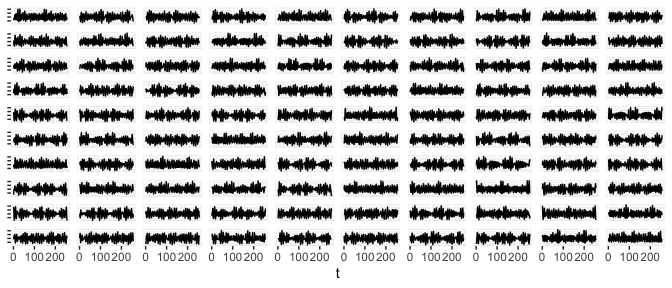100 realizations of x1.

A realization of $$x_1$$ looks like:

plot(m1[,1], type = "l", ylim = c(-5, 5), xlab = "t", ylab = "")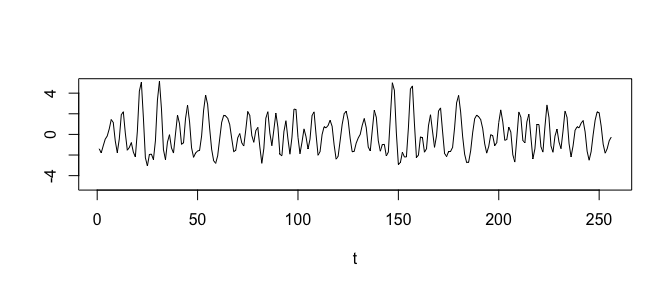The 256 time points in the first realization of x1.

Its spectrum estimation shows peaks at six frequencies as expected:

spectrum(m1)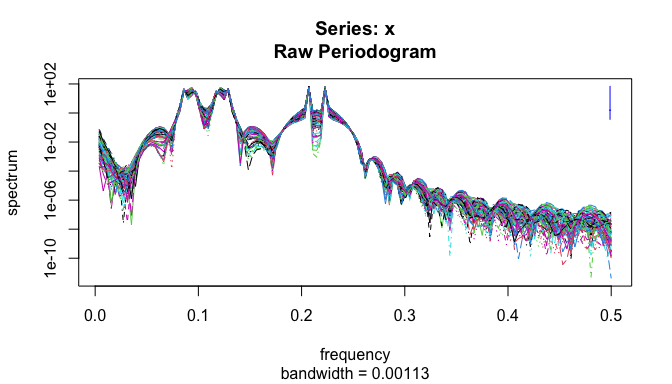The spectrum estimation of x1.

Now we approximate $$x_1$$’s bicoherence by bicoherence:

bc1 <- bicoherence(m1)

… and define an R function to plot the heat map of the estimated bicoherence:

heatmap_bicoherence <- function(bc) {
ggplot(bc) +
geom_raster(aes(f1, f2, fill = value)) +
coord_fixed() +
scale_alpha(guide = "none")
}

heatmap_bicoherence(bc1)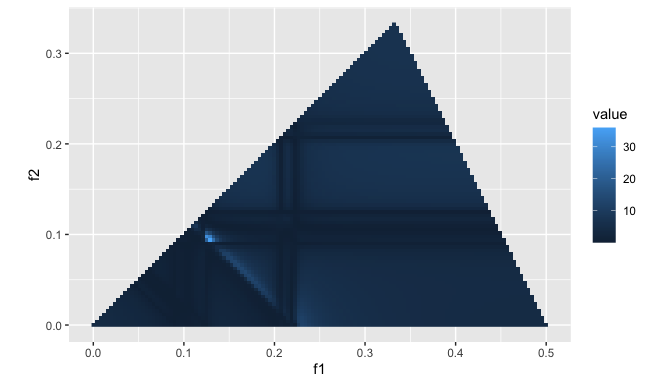x1’s estimated bicoherence.

Note that the highest peak is at the bifrequency $$(f_1, f_2) = (\frac{\lambda_5}{2 \pi}, \frac{\lambda_4}{2 \pi}) \approx (0.127, 0.095)$$.

# A three-channel model of quadratic signal processing

Another example of QPC consists of three channels, which accept series of periodic input signals and suffer from Gaussian noises. From a couple of the channels, say $$C_1$$ and $$C_2$$, we observe the summation of input and noises as their output. On the other hand the last channel, called $$C_3$$, adds $$C_1$$’s output multiplied by $$C_2$$’s to its own input and noise.

Here, assume that $$C_1$$’s input is a triangle wave of a fixed frequency with varying initial phases. A rectangle wave of another frequency for $$C_2$$’s input. A cosinusoidal curve of yet another frequency for $$C_3$$’s input. Running the following code simulates the model.

Fcoef1 <- 1.2
Fcoef2 <- 0.7
Fcoef3 <- 0.8

i1 <- function(x, p) {2 * asin(sin(Fcoef1 * x + p))}
i2 <- function(x, p) {ifelse(cos(Fcoef2 * x + p) >= 0, -1, 1)}
i3 <- function(x, p) {cos(Fcoef3 * x + p)}

Qcoef <- 0.3

tc <- function(k) {
set.seed(k)
ps <- runif(3, min = 0, max = 2*pi)
function(x) {
c1 <- i1(x, ps) + rnorm(length(x), mean = 0, sd = 1)
c2 <- i2(x, ps) + rnorm(length(x), mean = 0, sd = 1)
c3 <- Qcoef * c1 * c2 +
i3(x, ps) + rnorm(length(x), mean = 0, sd = 1)
data.frame(c1, c2, c3)
}
}

N2 <- 100

sample_tc <- function() {
Map(function(f) {f(seq_len(256))}, Map(tc, seq_len(N2)))
}

c1_data_frame <- function(y) {
do.call(cbind, Map(function(k) {y[[k]]$c1}, seq_len(N2))) } c2_data_frame <- function(y) { do.call(cbind, Map(function(k) {y[[k]]$c2}, seq_len(N2)))
}

c3_data_frame <- function(y) {
do.call(cbind, Map(function(k) {y[[k]]\$c3}, seq_len(N2)))
}

y1 <- sample_tc()
d1 <- c1_data_frame(y1)
d2 <- c2_data_frame(y1)
d3 <- c3_data_frame(y1)

That is, we obtain 100 series of data with 256 points. To be specific, $$C_1$$’s triangle wave has cycle $$\frac{2 \pi}{1.2}$$, the cycle of $$C_2$$’s rectangle wave is $$\frac{2 \pi}{0.7}$$, and the one of $$C_3$$’s cosinusoidal wave is $$\frac{2 \pi}{0.8}$$.

$$C_1$$’s output looks like:

plot(d1[,1], type = "l", ylim = c(-5, 5), xlab = "t", ylab = "")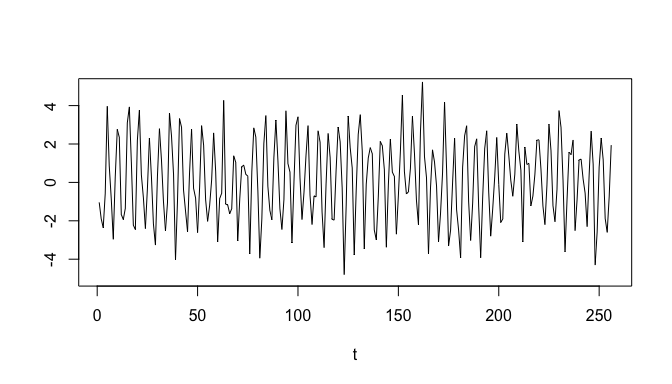A sample path of d1.

And $$C_2$$’s output:

plot(d2[,1], type = "l", ylim = c(-5, 5), xlab = "t", ylab = "")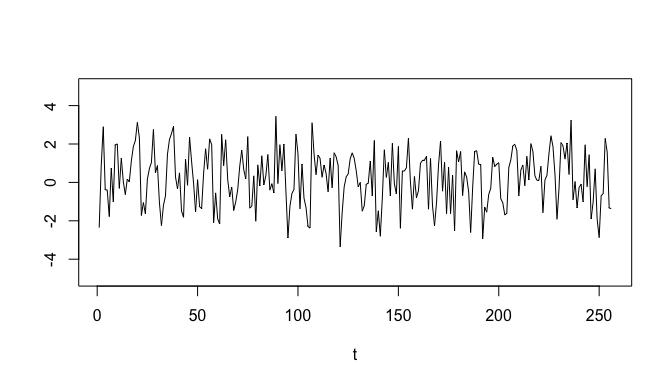A sample path of d2.

$$C_3$$’s output:

plot(d3[,1], type = "l", ylim = c(-5, 5), xlab = "t", ylab = "")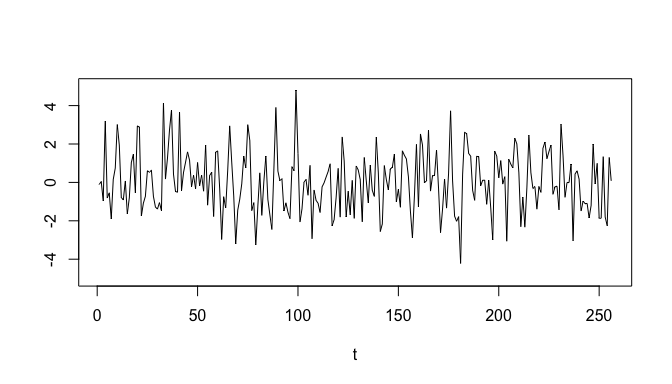A sample path of d3.

Their spectrum estimation show significant peaks at expected frequencies:

spectrum(d1)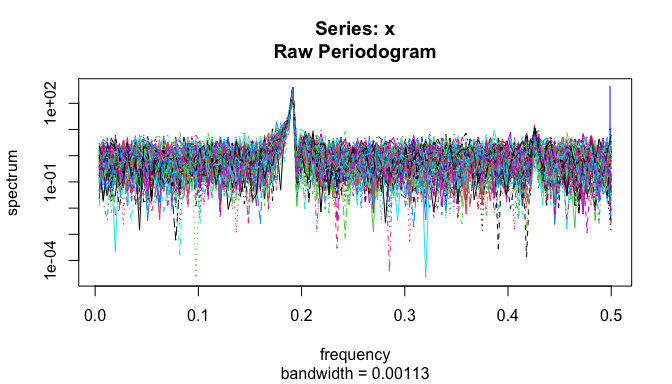The spectrum estimation of C1.

spectrum(d2)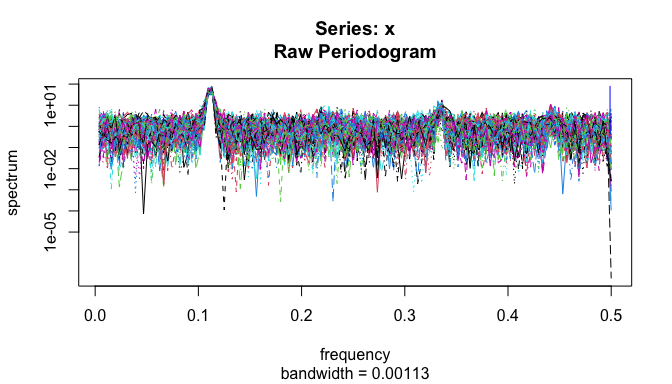The spectrum estimation of C2.

spectrum(d3)